## Example Questions

1 2 3 4 5 6 7 8 9 10 12 Next →

### Example Question #25 : Ordering

Order these decimals from greatest to least: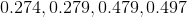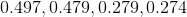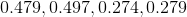Explanation:

Ordering decimals from greatest to least means we list them from the biggest number to the smallest number. When doing this, line up each of your numbers by their decimal points. We look at the tenths place first and order them according to that number. Then move on to the hundredths place and so on until each of the numbers are lined up from greatest to smallest. For this problem that would look like this: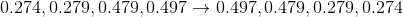### Example Question #29 : How To Order Decimals From Least To Greatest Or From Greatest To Least

Order these decimals from greatest to least: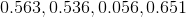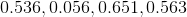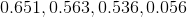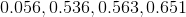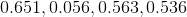Explanation:

Ordering decimals from greatest to least means we list them from the biggest number to the smallest number. When doing this, line up each of your numbers by their decimal points. We look at the tenths place first and order them according to that number. Then move on to the hundredths place and so on until each of the numbers are lined up from greatest to smallest. For this problem that would look like this: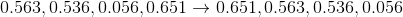### Example Question #451 : Arithmetic

Order these decimals from greatest to least: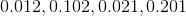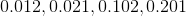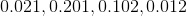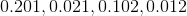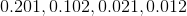Explanation:

Ordering decimals from greatest to least means we list them from the biggest number to the smallest number. When doing this, line up each of your numbers by their decimal points. We look at the tenths place first and order them according to that number. Then move on to the hundredths place and so on until each of the numbers are lined up from greatest to smallest. For this problem that would look like this: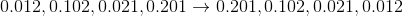### Example Question #451 : Arithmetic

Order these decimals from greatest to least: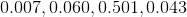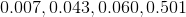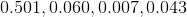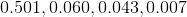Explanation:

Ordering decimals from greatest to least means we list them from the biggest number to the smallest number. When doing this, line up each of your numbers by their decimal points. We look at the tenths place first and order them according to that number. Then move on to the hundredths place and so on until each of the numbers are lined up from greatest to smallest. For this problem that would look like this: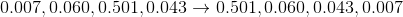### Example Question #31 : How To Order Decimals From Least To Greatest Or From Greatest To Least

Order these decimals from greatest to least: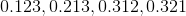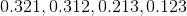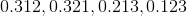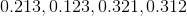Explanation:

Ordering decimals from greatest to least means we list them from the biggest number to the smallest number. When doing this, line up each of your numbers by their decimal points. We look at the tenths place first and order them according to that number. Then move on to the hundredths place and so on until each of the numbers are lined up from greatest to smallest. For this problem that would look like this: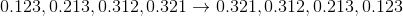1 2 3 4 5 6 7 8 9 10 12 Next →

### All SAT Math Resources